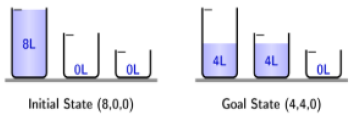# Artificial Intelligence - Approaches to Artificial Intelligence

>>>>>>>>Approaches to Artificial Intelligence

Water jug puzzle: An eight-liter jug is filled with water, you are required to divide it into 4 + 4 liters. You may use two empty jugs of size 5 and 3 liters for this purpose. There is no other way of measuring water except by the size of the jugs, i.e., one can either empty a jug into another or fill another jug to its brimModel this puzzle as a state-space search problem. A state is represented by a tuple (A,B,C), where A is the amount of water in 8L jug, B is the amount of water in 5L jug and C is the amount of water in 3L jug.
The initial state is (8,0,0), if you transfer water from 8L jug to 5L jug we reach (3,5,0) state, and if you transfer the remaining water from 8L jug to 3L jug we reach (0,5,3) state. Now we can reverse these two transfers. The state-space expresses all valid states and their transitions. Build the state-space to answers the following questions.

• A

5• B

4• C

6• D

7Water jug puzzle: An eight-liter jug is filled with water, you are required to divide it into 4 + 4 liters. You may use two empty jugs of size 5 and 3 liters for this purpose. There is no other way of measuring water except by the size of the jugs, i.e., one can either empty a jug into another or fill another jug to its brimModel this puzzle as a state-space search problem. A state is represented by a tuple (A,B,C), where A is the amount of water in 8L jug, B is the amount of water in 5L jug and C is the amount of water in 3L jug.
The initial state is (8,0,0), if you transfer water from 8L jug to 5L jug we reach (3,5,0) state, and if you transfer the remaining water from 8L jug to 3L jug we reach (0,5,3) state. Now we can reverse these two transfers. The state-space expresses all valid states and their transitions. Build the state-space to answers the following questions.

• A

5• B

6• C

7• D

8Water jug puzzle: An eight-liter jug is filled with water, you are required to divide it into 4 + 4 liters. You may use two empty jugs of size 5 and 3 liters for this purpose. There is no other way of measuring water except by the size of the jugs, i.e., one can either empty a jug into another or fill another jug to its brimModel this puzzle as a state-space search problem. A state is represented by a tuple (A,B,C), where A is the amount of water in 8L jug, B is the amount of water in 5L jug and C is the amount of water in 3L jug.
The initial state is (8,0,0), if you transfer water from 8L jug to 5L jug we reach (3,5,0) state, and if you transfer the remaining water from 8L jug to 3L jug we reach (0,5,3) state. Now we can reverse these two transfers. The state-space expresses all valid states and their transitions. Build the state-space to answers the following questions.

• A

1, 2, 3 and 4• B

2,3 and 5• C

2 and 3• D

All are true• A

1 and 2• B

2 and 3• C

1, 2 and 3• D

None of the above• A

2 and 3• B

1 and 3• C

1 and 2• D

None of the above## Description

Syllabus Covered in this section is

Approaches to AI: Turing Test and Rational Agent Approaches; State Space Representation
of Problems, Hellllstic Search Techniques, Game Playing, Min-Max Search, Alpha Beta
Cutoff Procedures.

• Approaches to ai Questions can be used to give quizzes by any candidate who is preparing for UGC NET Computer Science
• Approaches to ai Questions can be used in the preparation of JRF, CSIR, and various other exams.
• Approaches to ai Questions can be used to gain a credit score in various undergraduate and postgraduate courses like BSc, MSc, and MCA
• This approaches to ai Questions section will help you test your analytical skills in a tricky method, thereby giving you an edge over other students
• Any student who wants to prepare for DOEACC A Level, DOEACC B Level, and DOEACC C level can also use these Objective Type Questions Answer.
• All candidates who have to appear for the Kendriya Vidyalaya Entrance exam can also refer to this mcq section.
• You can also get access to the approaches to ai MCQ ebook.